#### Illustrated Glossary » Transmission Lines

Transmission lines carry electric energy from one point to another in an electric power system. They can carry alternating current or direct current or a system can be a combination of both. Also, electric current can be carried by either overhead or underground lines. The main characteristics that distinguish transmission lines from distribution lines are that they are operated at relatively high voltages, they transmit large quantities of power and they transmit the power over large distances.

The types of transmission lines are:

Overhead AC transmission lines share one characteristic; they carry 3-phase current. The voltages vary according to the particular grid system they belong to. Transmission voltages vary from 69 kv up to 765 kv. The following are examples of different overhead transmission line structures in use today. The DC voltage transmission tower has lines in pairs rather than in threes (for 3-phase current) as in AC voltage lines. One line is the positive current line and the other is the negative current line.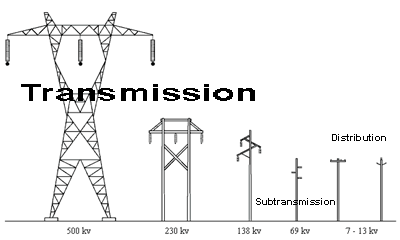Figure 1. Some typical transmission line structures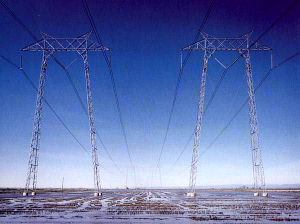Figure 2. Transmission lines crossing the San Fernando Valley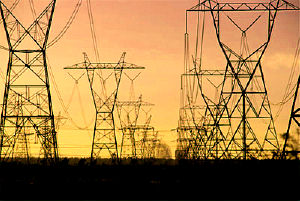Figure 3. 345 kv transmission lines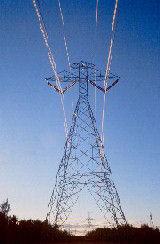Figure 4. DC voltage transmission lines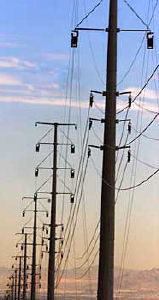Figure 5. Transmission lines above - subtransmission below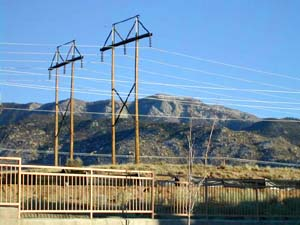Figure 6. 230 kv wood transmission line towers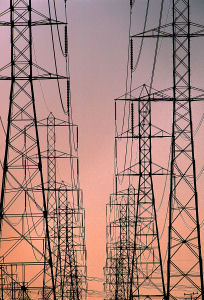Figure 7. Double set of transmission lines side by side

• The Lineman's and Cableman's Handbook, Shoemaker, T. M., Mack, J. E., Tenth Edition 2002, McGraw-Hill.

Subtransmission lines carry voltages reduced from the major transmission line system. Typically, 34.5 kv to 69 kv, this power is sent to regional distribution substations. Sometimes the subtransmission voltage is tapped along the way for use in industrial or large commercial operations. Some utilities categorize these as transmission lines.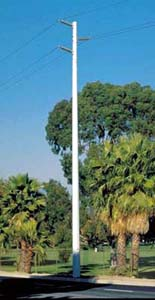Figure 8. Subtransmission lines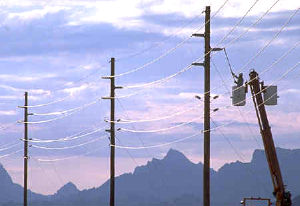Figure 9. Subtransmission lines above and distribution lines below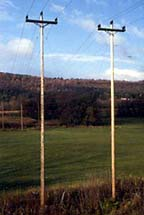Figure 10. 46 kv subtransmission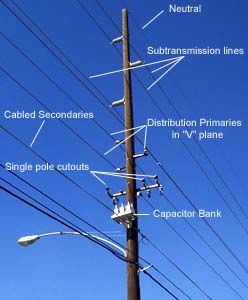Figure 11. Subtransmission lines with distribution primaries and secondaries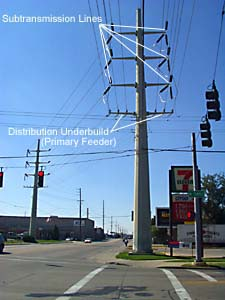Figure 12. Subtransmission lines with distribution underbuild

Underground transmission lines are more common in populated areas. They may be buried with no protection, or placed in conduit, trenches, or tunnels.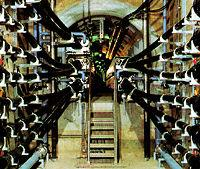Figure 13. Transmission lines are installed in a tunnel, which enables many circuits in a limited area.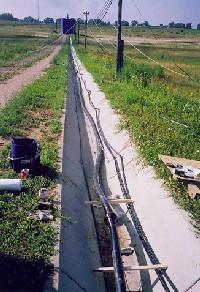Figure 14. Transmission line laid in a trench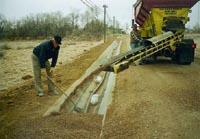Figure 15. Covering the transmission line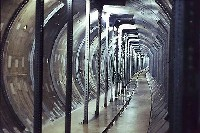Figure 16. Underground transmission line tunnel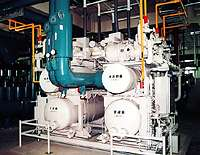Figure 17. Heat is generated when electricity flows through cables, limiting the power transmission capacity in tunnels. To increase the capacity, a tunnel cooling system can be installed. The system above circulates cold water through the tunnels.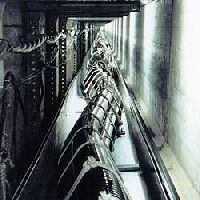Figure 18. Cable snaking through underground transmission tunnel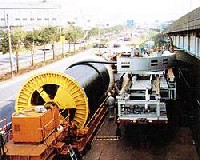Figure 19. Cable installation of underground transmission line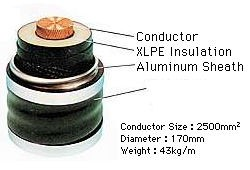Figure 20. Cross section of underground transmission line